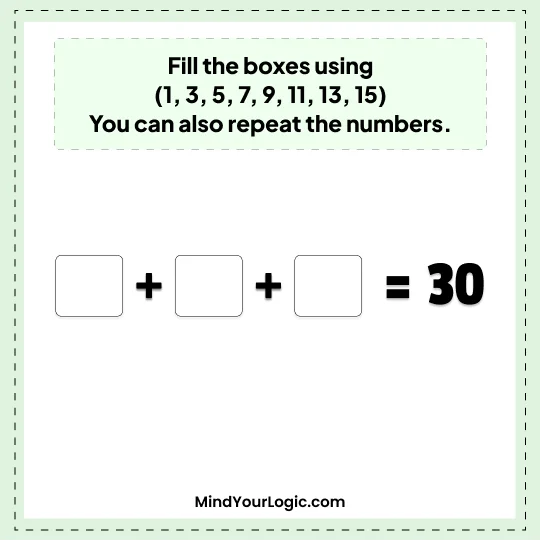# Fill the boxes - Math Riddle

###### 109.Math Riddles
```Fill the boxes using (1, 3, 5, 7, 9, 11, 13, 15)
You can also repeat the numbers```• `30`
```Explanation :

There can be multiple solutions to this problem.
Listing three of them
A) 11+13+6(rotating 9) = 30
B) 11+13+3! = 30
c) 11.5+5.5+13 = 30```